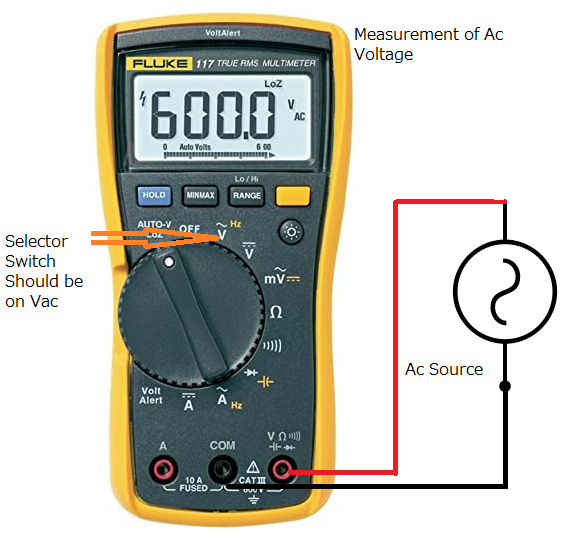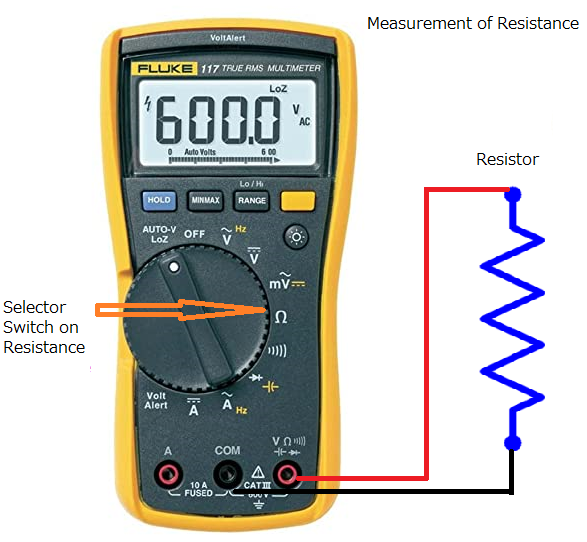Mutlimeter is a very useful electronics device which help to measure different electrical as well as electronic parameters e.g. Voltage,Current,Resistance etc.In this article we will discuss different functions of multimeter.So firstly to understand the multimeters funstions we should understand the working of multimeter and

Important Sections of MultiMeter:

Display:

Selector Switch:

Ports:
• Voltage/Ohms
• Common
• Current
Probes

The second probe we connect to another port, we connect whatever quantity we want to measure.
Ports:

When we measure currents then current port is used.With the help of V / Ω, we measure the voltage and resistance and test the continuity. These ports depend on the multimeter and may vary.

Functions of Multimeter:

• Ac Voltage/Frequency
• Dc Voltage
• milli Volts Ac/Dc
• Resistance
• Continuity
• Diode/Capacitance
• Ac Current
• Dc Current
• Auto Switch
• Hold Switch
• Functions Switch
• Rotary Switch

Ac Voltage/Frequency:

This function allow us to measure Ac Voltage of any circuit and Frequency of any signal.The meaured  range of voltage and  frequency depends up on the multimeter operating range as per manufacturing specification.

Dc Voltage:

With help of this function we measure Dc voltage of any circuit and analyse the circuit condition as per our requirement.We measure the voltage of Dc battery,cell etc.

milli Voltage Dc/Ac:

This function allow us to measure the low voltage ranges such as milli volts,micro volts etc.This function is introduce beacause at high voltage range function the measured value should not be as accurate as milliVolt function.

Resistance:

This function allow us to measure the resistance across any circuit.This function denoted by ohms symbol as seen in picture above.The range of  measured resistance should be selected from the Range switch.

Continuity:

With help of this function we can check the continuity between any two circuit nodes or meausre the continuity of any component e.g. Diode in forword Bias, Zero ohms resistance.

Diode/Capacitance:

This function allow us to check functionality of diode and we can check diode with help of this function.With help of this function we can also measure the capacitance of any circuit or capacitor.

Ac current/Dc current:

This functions allow us to make the meausrement of Ac current and Dc current by putting multimeter in series with the circuit.We should take care while measure current beacause if connections are not ok it will result in burning of current fuse.

Auto Switch:

Auto switch enable automatic detection of incoming signal and set the function automatically which is inputed.We should select this swtich before measuring any parameter.

Hold Swtich:

This switch enable us to hold the measured value  on seven segment display.

Function Switch:

Function switch in digital multimeter set the paramter to be measured and only enable the parameter which we want to measure.

Friends after discussion of functions of digital multimeter now we should also discuss how to use the multimeter for measurement.

Measurement of Dc Voltage:

To measure the Dc voltage with help of Digital multimeter firstly set the selector switch on Dc voltage.Now connect the Voltage/ohms lead to + end of cell and common lead to - end of cell as described in below picture.

Measurement of Ac Voltage:

For measurement of Ac voltage firstly set the selector switch to Vac and after that connect connection leads to ac source whose voltage is need to measured.Please make your connection as per below picture.Measurement of  Resistance:

For measuring the resistor of any resistor or resistance of any circuit firstly select the selector switch on reisitance and connect the leads as per below picture.Continuity Check:

For continuity check set selector switch on continuity and connect the leads as per beow picture.If circuit have very low resistance then digitsl multimeter create beep sound.

Ac current Measurement:

For the measurement of Ac current firstly set selector switch to Ac current and then connect the leads as per the below picture (in series with circuit).Note please make connection tight and correct for correct measurement.